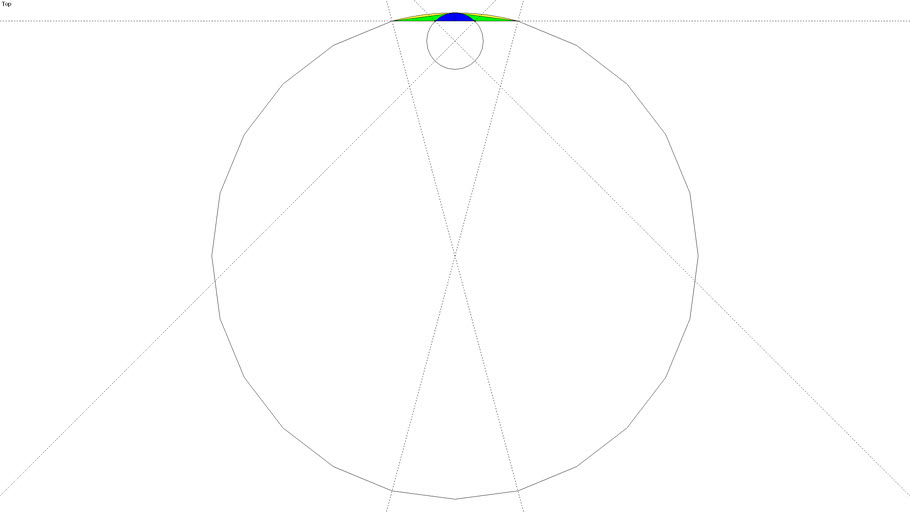# Arc Examples - Equal Bulge [How far out the arc bulges]

wmray
length = radius x sin (angle/2) x 2 bulge = radius x (1 - cos (angle/2)) length / bulge = ( sin (angle/2) x 2 ) / (1 - cos (angle/2)) #circle #example #trig #trigonemtry #tutorial
• Comments
Default Title